## Collision Process in Gases:

An electrical discharge is normally created from unionised gas by Collision Process in Gases. These processes are mainly gas processes which occur due to the collision between the charged particles and gas atoms or molecules.

These are of the following two types.

### Elastic collisions:

Elastic collisions are collisions which when occur, no change takes place in the internal energy of the particles but only their kinetic energy gets redistributed. These Collision Process in Gases do not occur in practice. When electrons collide with gas molecules, a single electron traces a zig-zag path during its travel. But in between the collisions it is accelerated by the electric field. Since electrons are very light in weight, they transfer only a part of their kinetic energy to the much heavier ions or gas molecules with which they collide. This results in very little loss of energy by the electrons and therefore electrons gain very high energies and travel at a much higher speed than the ions. Therefore in all electrical discharges electrons play a leading role.

Inelastic collisions: Inelastic collisions, on the other hand, are those in which internal changes in energy take place within an atom or a molecule at the expense of the total kinetic energy of the colliding particle. The Collision Process in Gases often results in a change in the structure of the atom. Thus all Collision Process in Gases that occur in practice are inelastic collisions. For example ionisation, attachment, excitation, recombi­nation are inelastic collisions.

### Mobility of Ions and Electrons:

When an ion moves through a gas under the influence of a static uniform electric field, it gains energy from the field between collisions and loses energy during collisions. Electric force on an electron/ion of charge e is eE, with the resulting acceleration being eE/m. When the energy gained by the ions from the electric field is small compared with the thermal energy, the drift velocity in the field direction Wi is proportional to the electrical field intensity E and may be expressed as follows: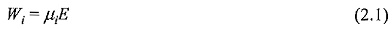where μi is called the mobility of ions. The mobility is mainly a characteristic of the gas through which the ion moves. At normal temperatures and pressures the mobility μ is of the order of several cm2/volt-sec.

However, the concept of ionic mobility cannot be directly applied to electrons because of their extremely low mass. Any externally applied electric field will cause the electrons to gain energies much higher than their mean thermal energy. So the electron drift velocity, which has been defined as the average velocity, with which the centre of mass of the electron swarm moves in the direction of the field, is not a simple function of E/p, but is determined from the energy distribution function. From the kinetic theory the electron drift velocity We is given in microscopic terms as follows: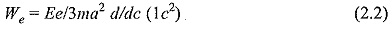where 1 is an equivalent mean free path of an electron with speed c.

### Diffusion Coefficient:

When particles possessing energy, which is exhibited as a random motion, are distributed unevenly throughout a space, then they tend to redistribute themselves uniformly throughout the space. This process is known as diffusion and the rate at which this occurrence is governed by the diffusion passing through unit area in unit time perpendicular to the concentration gradient and for unit concentration gradient. In three dimensions this may be written as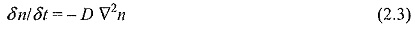where n is the concentration of particles.

Kinetic theory gives D in microscopic terms as follows: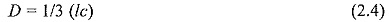where l is the mean free path and c the random velocity, the average being taken over c.

In electrical discharges, whenever there is a non-uniform concentration of charges there will be migration of these charges from regions of higher concentration to regions of lower concentration. This process is called diffusion and this causes a de-ionising effect in the regions of lower concentration. The presence of walls confining a given volume increases the de-ionisation effect since charged particles lose their charge on hitting the wall. Both diffusion and mobility result in mass motion described by a drift velocity caused either by unbalanced collision forces (concentration gradient) or by the electric field itself.

### Electron Energy Distributions:

For the development of a complete theory giving the relationship between the data concerning single Collision Process in Gases of electrons with gas molecules, and the experimentally obtained average properties of discharges, a knowledge of the electron energy distribution functions is essential. The most widely used distribution functions are the Maxwellian and Druyesteynian distributions which apply specifically to elastic conditions.

The Maxwellian distribution has been found to apply where there is thermal equilibrium between the electrons and molecules.

The distribution takes the form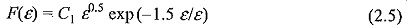where C1 is the constant and ε is the mean energy.

Druyesteynian distribution applies when the electron or ion energy is much greater than the thermal energy and is therefore expected to be more of application in transcend discharges. This distribution takes the form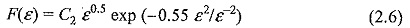where C2 is another constant.

### Collision Cross Section:

Collision cross section is defined as the area of contact between two particles during a collision. In other words, the total area of impact. This area of contact is different for each types of collision. For example, the area of impact is more for ionisation while for an excitation it is less. For simultaneously, occurring processes such as ionization, excitation, charge transfer, chemical reactions, etc., the effective cross section is obtained by simple a addition of all the cross sections. If qt is the total cross section, and if q, q, q… etc., are the cross sections for ionization, excitation, charge transfer, etc, respectively, then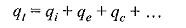Thus the use of collision cross sections instead of mean free paths has often proved to be advantageous. The collision cross section is also expressed in terms of the probability of a collision to take place, i.e.,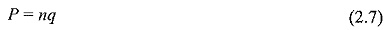which is the reciprocal of the mean free path.

### The Mean Free Path (λ):

The mean free path is defined as the average distance between collisions. When a discharge occurs large number of collisions occur between the electrons and the gas molecules. Depending on the initial energy of the colliding electron, the distance between the two collisions vary. The average of this is the mean free path. The free path is a random quantity and its mean value depends upon the concentration of particles or the density of the gas.

The mean free path can be expressed as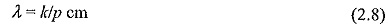where k is a constant and p is the gas pressure in microns.

The value of k for nitrogen is 5. From this equation it is seen that at a pressure of 1 torr, λ is 5 x 10-3 cm. If the pressure is 10-6 torr, then λ = 5 x 10+3 cm. From this it is seen that mean free path is very large at very low pressures and is very small at high pressures.

Scroll to Top